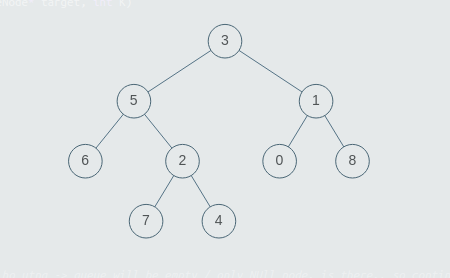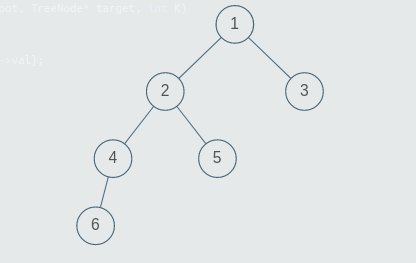New update is available. Click here to update.
Topics

# Special Binary Tree.

Easy0/40
Average time to solve is 15m## Problem statement

You are given an arbitrary binary tree. A binary tree is called special if every node of this tree has either zero or two children. You have to determine if the given binary tree is special or not.

If the given binary tree is special, return True. Else, return False to the given function.

Note:

``````1. A binary tree is a tree in which each node can have at most two children.
2. The given tree will be non-empty i.e the number of non-NULL nodes will always be greater than or equal to 1.
3. Multiple nodes in the tree can have the same values, all values in the tree will be positive.
``````
Detailed explanation ( Input/output format, Notes, Images )
Sample Input 1:
``````2
3 5 1 6 2 0 8 -1 -1 7 4 -1 -1 -1 -1 -1 -1 -1 -1
1 2 3 4 5 -1 -1 6 -1 -1 -1 -1 -1
``````
Sample Output 1:
``````True
False
``````
Explanation for sample input 1:``````Test Case1:

The tree given in Test Case 1, is shown in the image above. Note that nodes with the values 6, 7, 4, 0, 8, are leaf nodes and have no children, other nodes in the tree have two children each. So the given binary tree is special.
````````````Test Case 2:

The tree given in Test Case 2, is shown in the image above. Note that node with the value 4, has only one child, thus the given binary tree is not special.
``````
Sample Input 2:
``````2
5 2 3 8 1 -1 -1 7 9 -1 -1 5 6 -1 -1 -1 -1 -1 -1
1 5 7 -1 -1 6 3 9 8 -1 -1 -1 -1 13 -1 -1 -1
``````
Sample Output 2:
``````True
False
``````Console